# Python数据分析高薪实战第十天 EDA实战-全球新冠肺炎确诊病例趋势分析

### EDA 简介

EDA 区别于传统数据分析的重要一点就是，EDA 不做任何前置的假设，而是直接通过对原始数据进行分析，用可视化技术和各种统计的方法来探寻数据隐含的规律和信息。简单来说就是 从数据中寻找规律，而不是基于人工假设。

• 确定分析任务的目标；
• 筛选、清洗数据；
• 检测异常值与缺失值
• 数据分析，可视化；
• 挖掘特征之间的相互关系
• 挖掘特征与目标变量之间的关系
• 根据上一步的结果构建模型；
• 得出最终结论。

### 任务背景

[En]

Over the past year or so, the event that has had the greatest impact on us is the global pandemic of COVID-19. COVID-19 not only brings pain to patients, but also brings great challenges to the health care system. The essential reason why many patients are at risk is the shortage of medical resources. if the number of possible future visits can be predicted based on the existing confirmed data, then the government and hospitals will be able to plan and prepare medical resources in advance to greatly improve the medical environment of diagnosed patients.

[En]

Today we will try to predict the trend of confirmed cases in different countries over time based on one of the data sets.

### 数据集描述

train.csv 的格式如下：test.csv 的格式如下：### EDA 分析

#### 确定分析任务的目标

``````import pandas as pd
import matplotlib.pyplot as plt
import numpy as np
import seaborn as sns
import numpy as np
import random
from plotly import tools
import plotly.express as px
from plotly.offline import init_notebook_mode, iplot, plot
import plotly.figure_factory as ff
import plotly.graph_objs as go
``````

``````df_train = pd.read_csv("train.csv")

df_train
````````````df_test
``````#### 筛选、清洗数据

``````df_train.isna().sum()
``````

``````Id                    0
Province_State    20700
Country_Region        0
Date                  0
ConfirmedCases        0
Fatalities            0
dtype: int64
``````

``````df_train = df_train.fillna("")
df_train
````````````df_train.describe()
``````#### 数据分析、可视化

[En]

First of all, let's start from the national dimension and look at the proportion of historical diagnosis in different countries. Since the data in this data table is a table in which the proportion of diagnoses changes over time, we cannot aggregate the country dimension directly, which will take into account a lot of repetition.

• 首先按国家、省份、和日期维度聚合，并求和；
• 之后按国家和省份维度聚合，但聚合方式为取最大值；
• 最后按照国家维度聚合求和，并排序。

``````df_countries = df_train.groupby(["Country_Region","Province_State","Date"])["ConfirmedCases"].sum()
df_countries = df_countries.groupby(["Country_Region", "Province_State"]).max()
df_countries = df_countries.groupby(["Country_Region"]).sum().sort_values(ascending=False)

``````

``````fig = px.bar(df_countries, x=df_countries.index, y='ConfirmedCases', labels={'x':'Country'},
color="ConfirmedCases", color_continuous_scale=px.colors.sequential.Bluered)
fig.update_layout(title_text='国家历史最高确诊数')
fig.show()
``````[En]

It can be seen that the number of confirmed cases in the United States is much higher than that in other countries. We can check the situation in other countries except the United States by selecting the box.[En]

Even excluding the United States, the remaining countries are still very different. From these maps, it is not difficult to find that the country feature should be one of the core features in predicting the number of confirmed cases.

[En]

Next, let's take the United States as an example to analyze the changing trend of confirmed cases over time.

``````
df_usa_records = df_train.loc[df_train["Country_Region"]=="US", ["Province_State","Date", "ConfirmedCases", "Fatalities"]]

df_usa_records = df_usa_records.groupby("Date").sum()

df_usa_records = df_usa_records.reset_index()

df_usa_records
````````````fig = px.bar(df_usa_records,x='Date', y='ConfirmedCases', color="ConfirmedCases", color_continuous_scale=px.colors.sequential.Magma)
fig.update_layout(title_text='美国随时间确诊病例数')
fig.show()
````````````fig = px.bar(df_usa_records,x='Date', y='Fatalities', color="Fatalities", color_continuous_scale=px.colors.sequential.Magma)
fig.update_layout(title_text='美国随时间死亡病例数')
fig.show()
``````[En]

We can see that the overall trend is similar to the diagnosis curve, but compared with the diagnosis curve has a certain lag, but also basically in line with intuition.

``````df_brz_records = df_train.loc[df_train["Country_Region"]=="Brazil", ["Province_State","Date", "ConfirmedCases", "Fatalities"]]
df_brz_records = df_brz_records.groupby("Date").sum()
df_brz_records = df_brz_records.reset_index()
fig = px.bar(df_brz_records,x='Date', y='ConfirmedCases', color="ConfirmedCases", color_continuous_scale=px.colors.sequential.Magma)
fig.update_layout(title_text='巴西随时间确诊病例数')
fig.show()
````````````fig = px.bar(df_brz_records,x='Date', y='Fatalities', color="Fatalities", color_continuous_scale=px.colors.sequential.Magma)
fig.update_layout(title_text='巴西随时间死亡病例数')
fig.show()
``````[En]

As can be seen from the picture above, the number of diagnoses and deaths in Brazil is similar to that in the United States, and is still rising over time. But since late April, growth has been much steeper than in the United States. From here we can see that in addition to the differences in the total number of confirmed cases among different countries, there are also differences in the development of the number of confirmed cases, further indicating that the national dimension should be a core feature.

#### 特征工程

[En]

Now let's start to build the model, from the previous analysis, we can know that the time and country dimensions are important reference indicators. This is our core feature, but when we recall that the linear regression we used before often requires that the feature is a number, so that we can use the gradient algorithm to calculate the model, and our country is a category value, and time is a special type, what are we going to do? This requires us to preprocess the two features and convert them into numbers. This is the characteristic engineering link that we are familiar with.

（1）处理日期数据

[En]

The way to deal with date data is to convert the date into three numbers: year, month, and day, and create new fields. The logic and code of the processing are as follows:

``````
def get_year(date_str):
comps = date_str.split("-")
return int(comps)

def get_month(date_str):
comps = date_str.split("-")
return int(comps)

def get_day(date_str):
comps = date_str.split("-")
return int(comps)

df_train["Year"] = df_train.Date.apply(get_year)
df_train["Month"] = df_train.Date.apply(get_month)
df_train["Day"] = df_train.Date.apply(get_day)

df_train
``````（2）处理国家的特征

[En]

From the process of screening data, we know that there are many deficiencies in provincial processing. But there are still some records that have a value, so we can't discard this field directly, but if we use it directly as a feature, it may affect the result of the model.

``````df_train["Country_Region"] = df_train["Country_Region"] + df_train["Province_State"]
df_train["Country_Region"].value_counts()
``````

``````ChinaLiaoning                      115
Egypt                              115
Burundi                            115
USDelaware                         115
Panama                             115
...

ChinaJiangsu                       115
Congo (Kinshasa)                   115
FranceSaint Pierre and Miquelon    115
Colombia                           115
Name: Country_Region, Length: 313, dtype: int64
``````

[En]

As you can see, the records with provincial data have been spliced into the country field.

``````from sklearn.preprocessing import LabelEncoder
encoder = LabelEncoder()
df_train["Country_Region"] = encoder.fit_transform(df_train["Country_Region"])
df_train
``````（3）抽取训练特征和目标特征

``````df_train_final = df_train[["Country_Region", "Year", "Month", "Day"]]
labels = df_train.ConfirmedCases
``````

#### 模型训练

[En]

Now that our features are ready, we need to choose the appropriate model architecture to train our prediction model. In the previous course, we used a linear regression model, but linear regression does not apply to this scenario.

[En]

From the contents of our table above, we can see that the country is coded into a serial number. Although it is a number, the size of the number itself is meaningless. For example, 1 is Afghanistan, 2 is the United States, 3 is Panama, Afghanistan and Panama are very few, and the United States is very much. This is the so-called non-linear relationship, which simply means that the size of this value cannot be used as a basis for judgment. However, from the previous analysis, the country itself is critical to the development of the number of confirmed cases.

``````
from xgboost import XGBRegressor

xgb = XGBRegressor(n_estimators = 2500 , random_state = 0 , max_depth = 27)

xgb.fit(df_train_final, labels)
``````

``````XGBRegressor(base_score=0.5, booster='gbtree', colsample_bylevel=1,
colsample_bynode=1, colsample_bytree=1, gamma=0, gpu_id=-1,
importance_type='gain', interaction_constraints='',
learning_rate=0.300000012, max_delta_step=0, max_depth=27,
min_child_weight=1, missing=nan, monotone_constraints='()',
n_estimators=2500, n_jobs=8, num_parallel_tree=1, random_state=0,
reg_alpha=0, reg_lambda=1, scale_pos_weight=1, subsample=1,
tree_method='exact', validate_parameters=1, verbosity=None)
``````

#### 获取结论

``````df_test = df_test.fillna("")
df_test["Year"] = df_test.Date.apply(get_year)
df_test["Month"] = df_test.Date.apply(get_month)
df_test["Day"] = df_test.Date.apply(get_day)
df_test["Country_Region"] = df_test["Country_Region"] + df_test["Province_State"]
df_test
````````````df_test_final = df_test[["Country_Region", "Year", "Month", "Day"]]
df_test["predict_confirm"] = xgb.predict(df_test_final)
df_test
````````````df_train[df_train.Date >= "2020-04-02"]
``````### 小结

• EDA 的概念和基本的步骤。
• 遵循 EDA 的基本步骤来进行了新冠肺炎蔓延趋势的案例实战，主要包括：
• 通过 fillna 填充缺失数据；
• 通过多次 groupby 聚合来处理出我们希望要的数据；
• 通过 plotly 绘制柱状图来分析相关趋势；
• 通过对字段 apply 处理函数来拆分日期维度；
• 通过 LabelEncoder 来将国家处理为数值；
• 通过 xgboost 来拟合非线性关系的数据。

Original: https://blog.csdn.net/fegus/article/details/124584067
Author: 办公模板库 素材蛙
Title: Python数据分析高薪实战第十天 EDA实战-全球新冠肺炎确诊病例趋势分析

## Title: tensorflow安装步骤（CPU版本，Anaconda环境下，Windows10）

Anaconda安装步骤（Windows10）

## 一、TensorFlow2.3.0安装步骤

1.打开Anaconda Prompt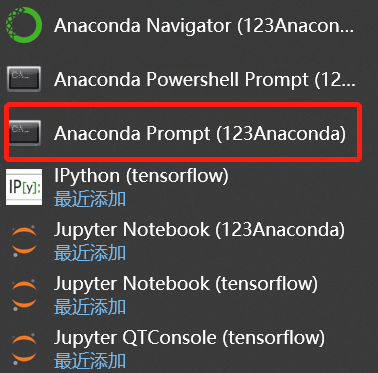2.输入下面命令，利用Anaconda创建一个python3.7的环境，环境名称为 tensorflow2（名称任意）

``````conda create -n tensorflow2 python=3.7
``````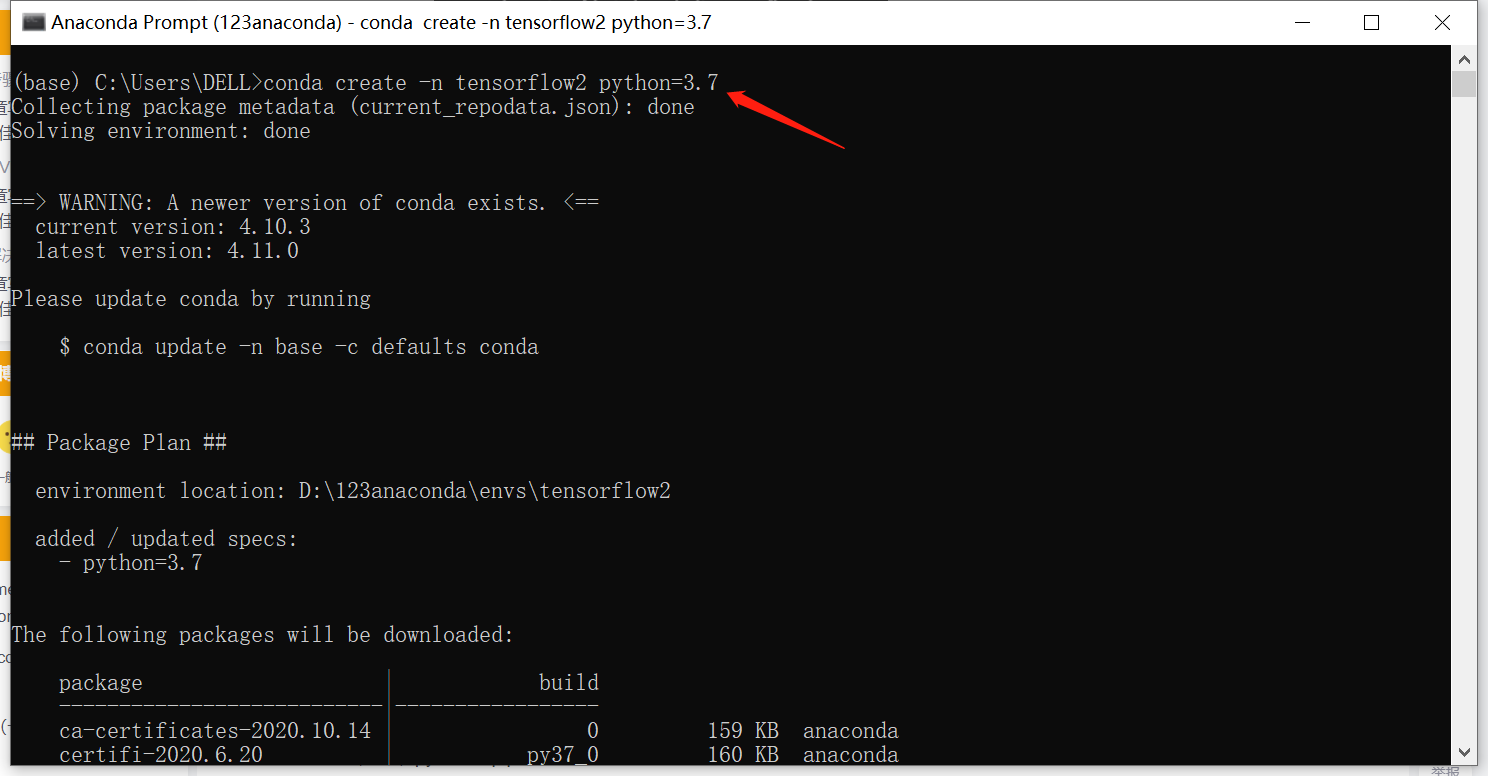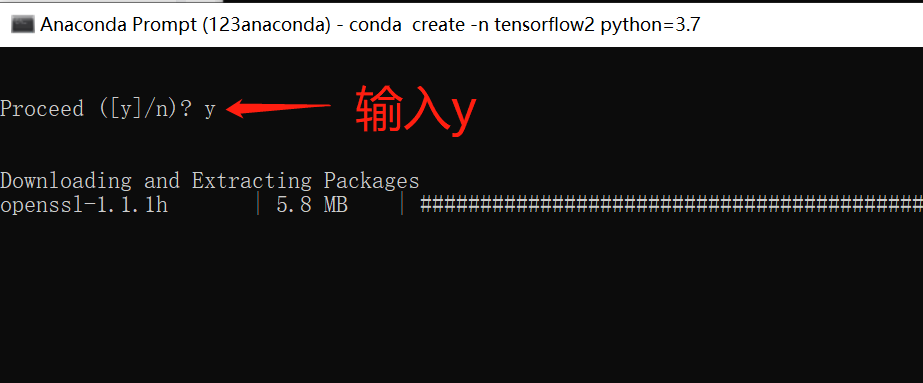3.输入下面命令，进入名称为 tensorflow2的环境中去（名称任意）

``````conda activate tensorflow2
``````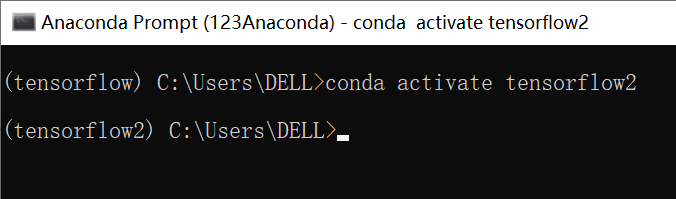4.输入下面命令，安装python的pip工具

``````python -m pip install --upgrade pip
``````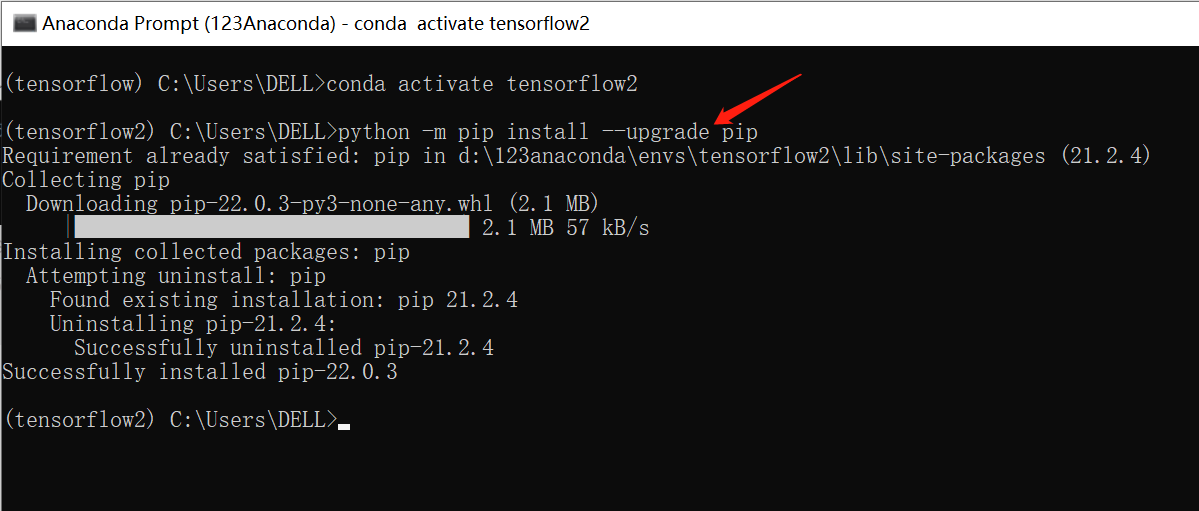5.输入下面命令，安装TensorFlow2.3.0
（切换到豆瓣源进行安装）

``````pip install tensorflow-cpu==2.3.0 -i https://pypi.douban.com/simple/
``````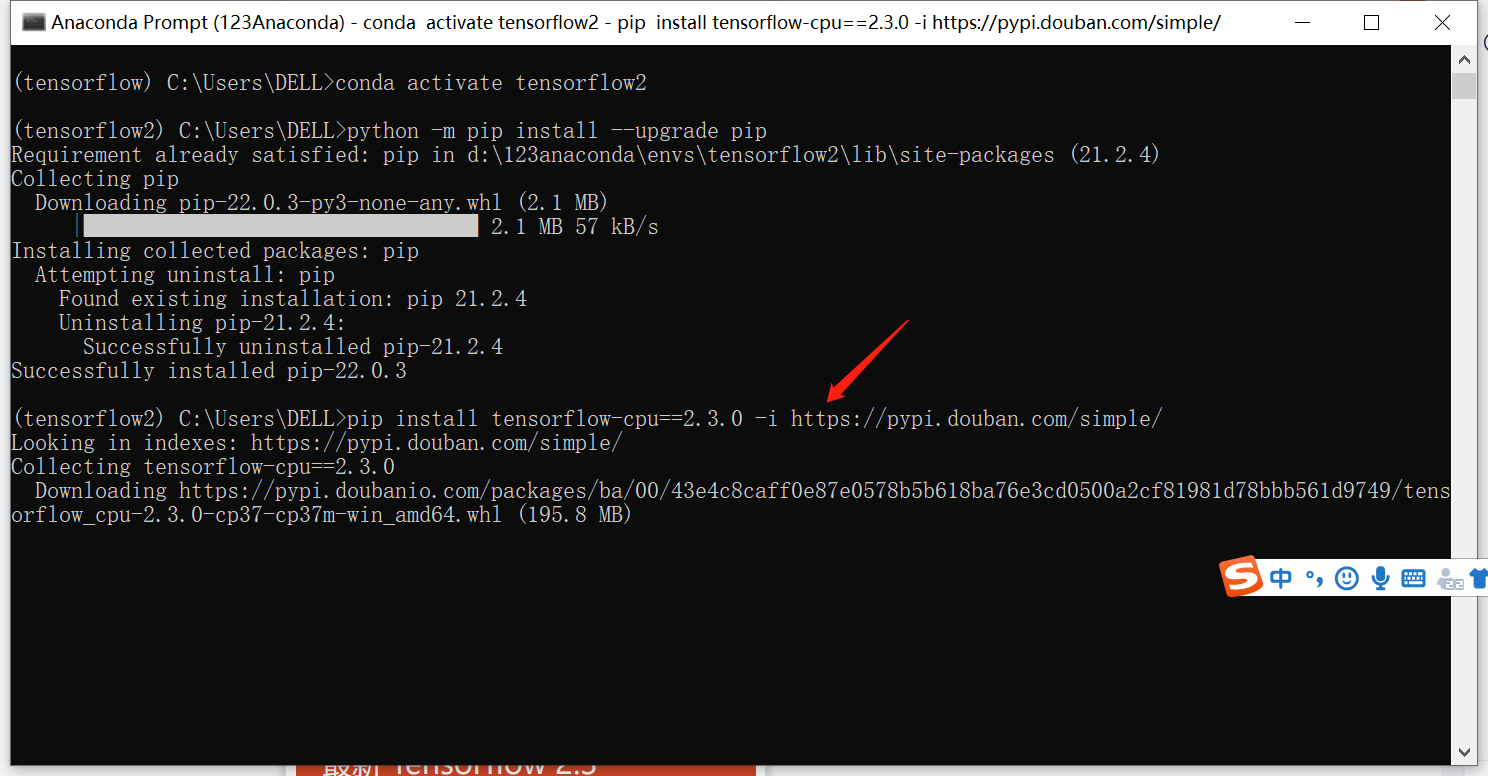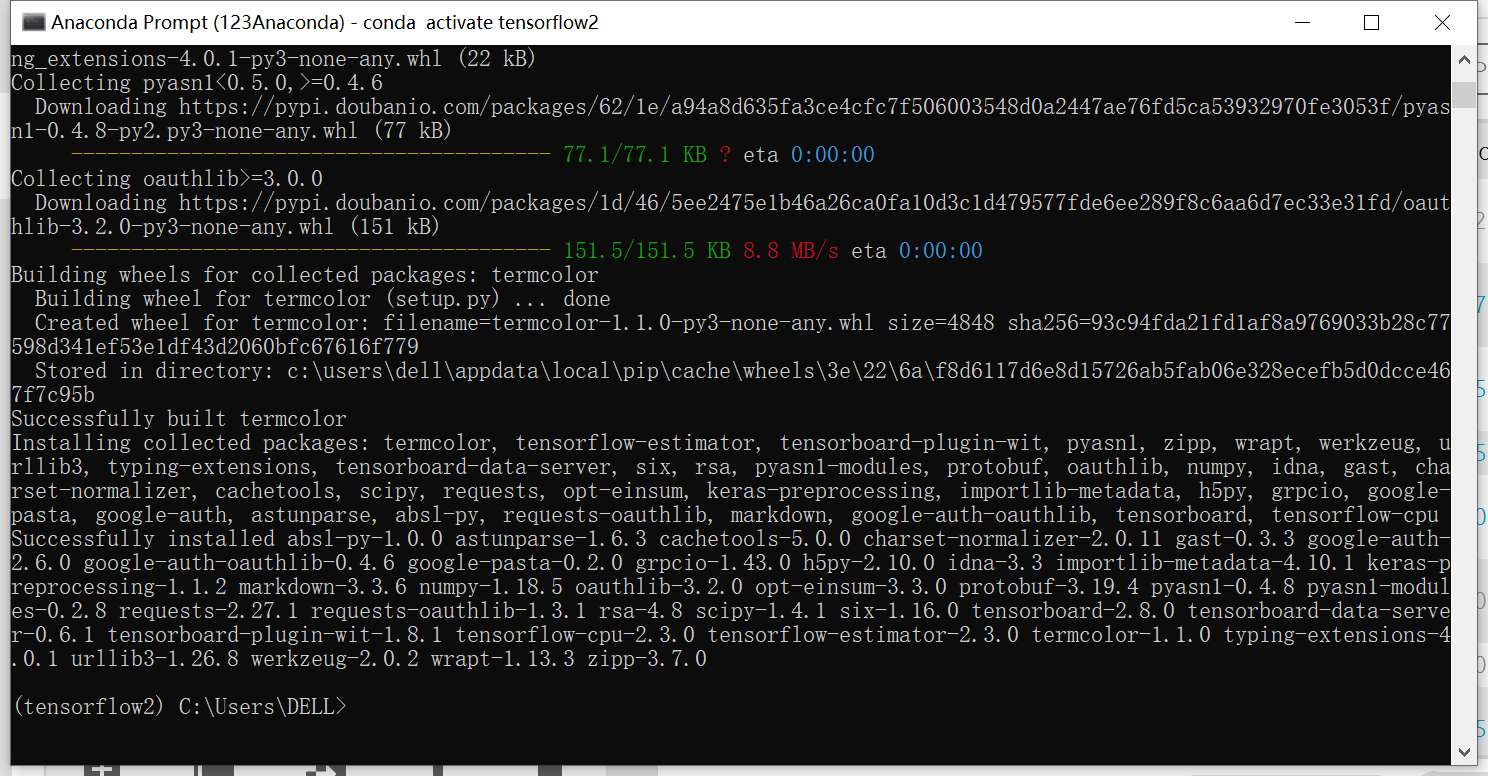## 二、对TensorFlow2.3.0进行验证

1.打开Anaconda Navigator2.点击 Environment，并选择刚刚建好的 tensorflow2环境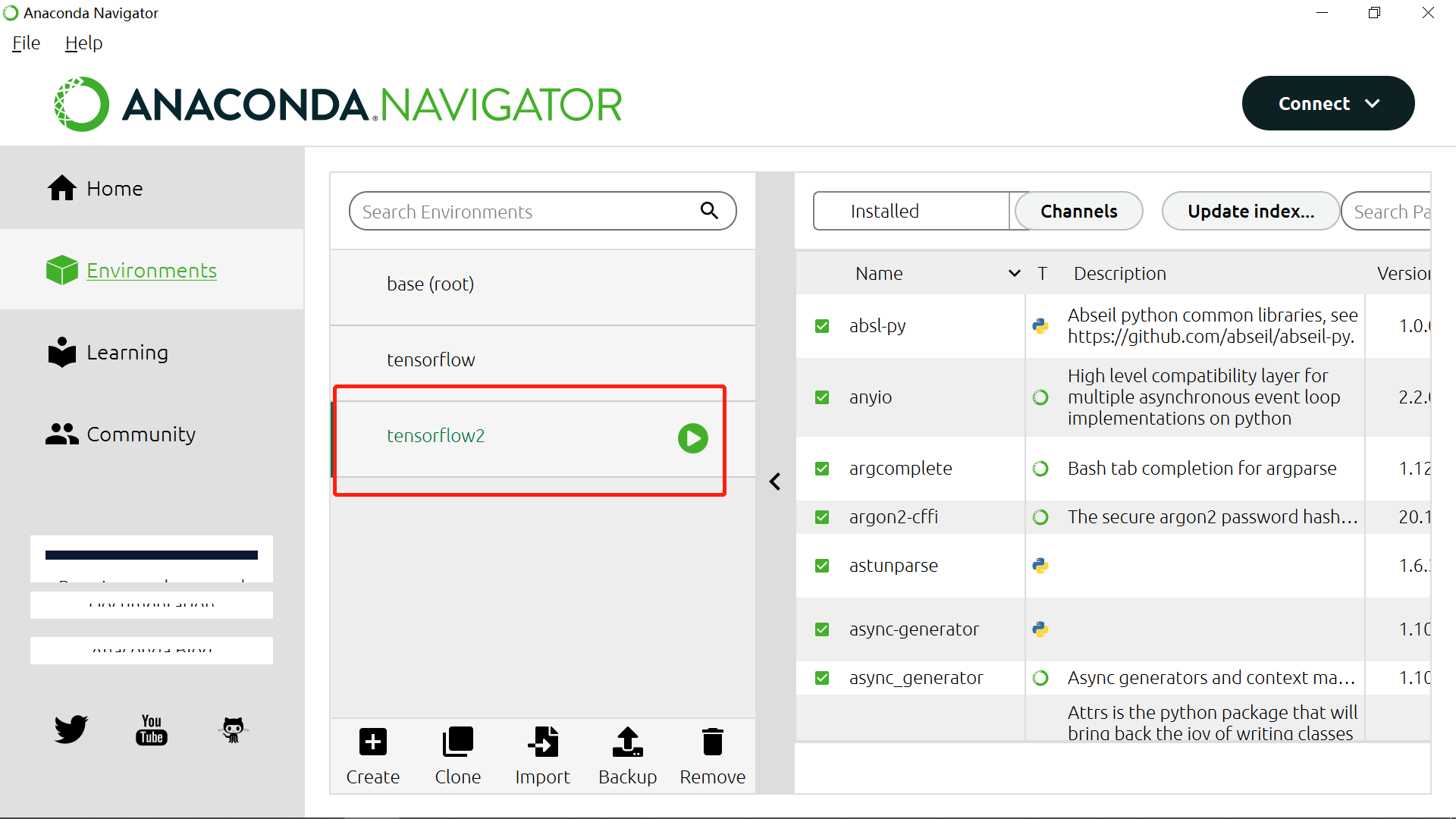3.回到主界面，找到JupyterLab，点击 Install，等待JupyterLab安装完成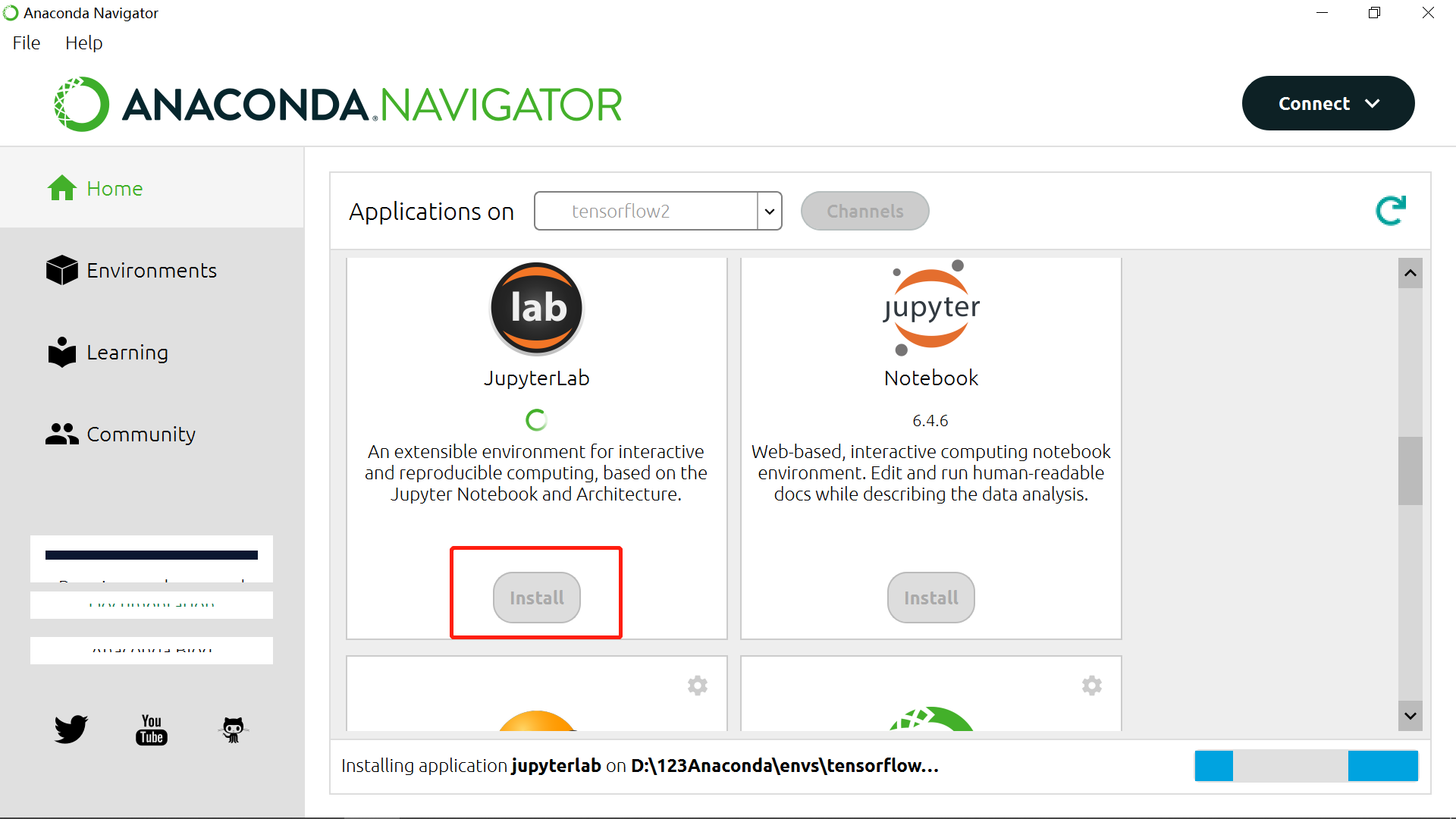3.JupyterLab安装完成后，点击 Launch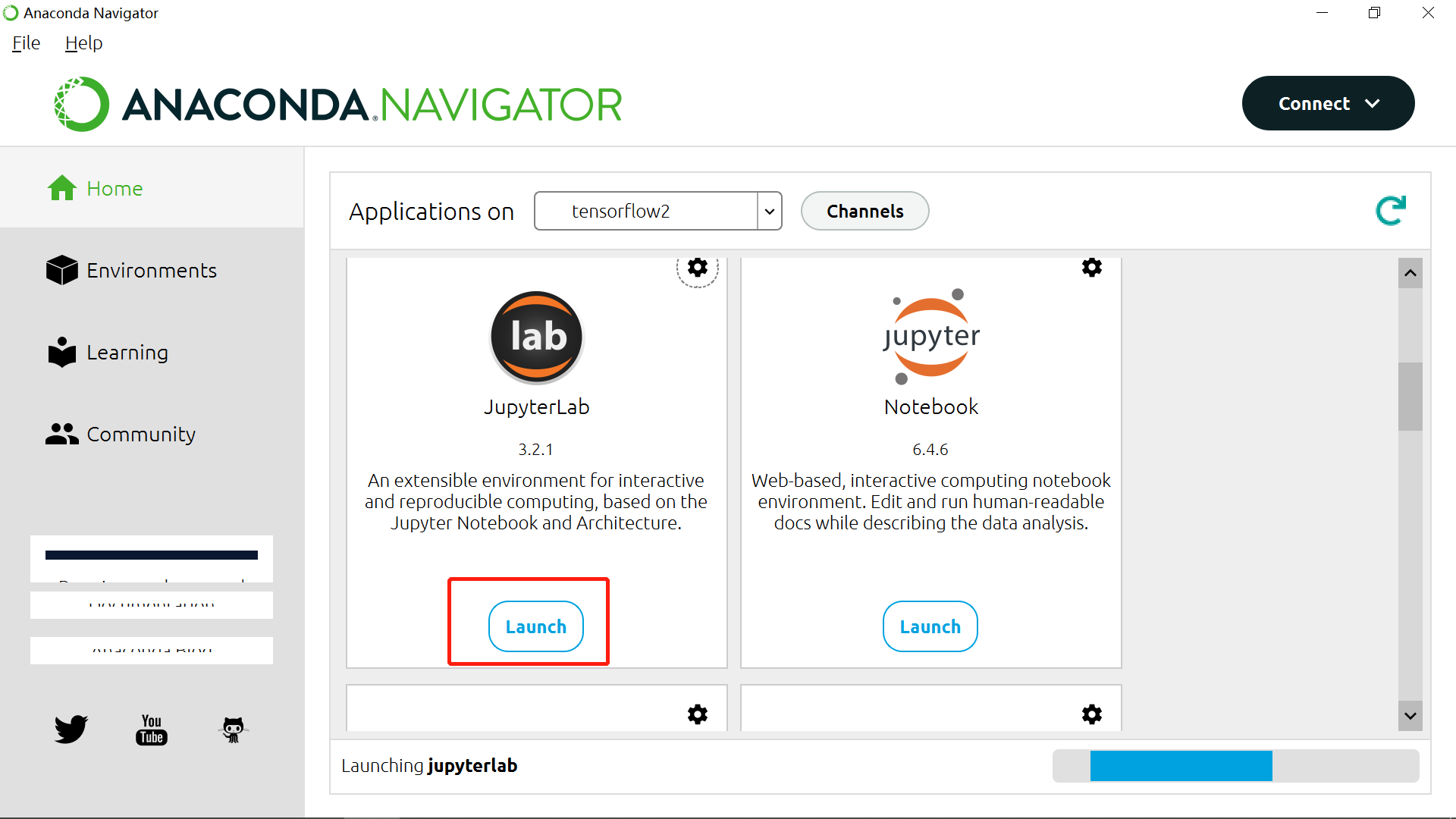4.进入JupyterLab页面后，新建一个python的file，输入两行程序并运行

``````import tensorflow as tf
print(tf.__version__)
``````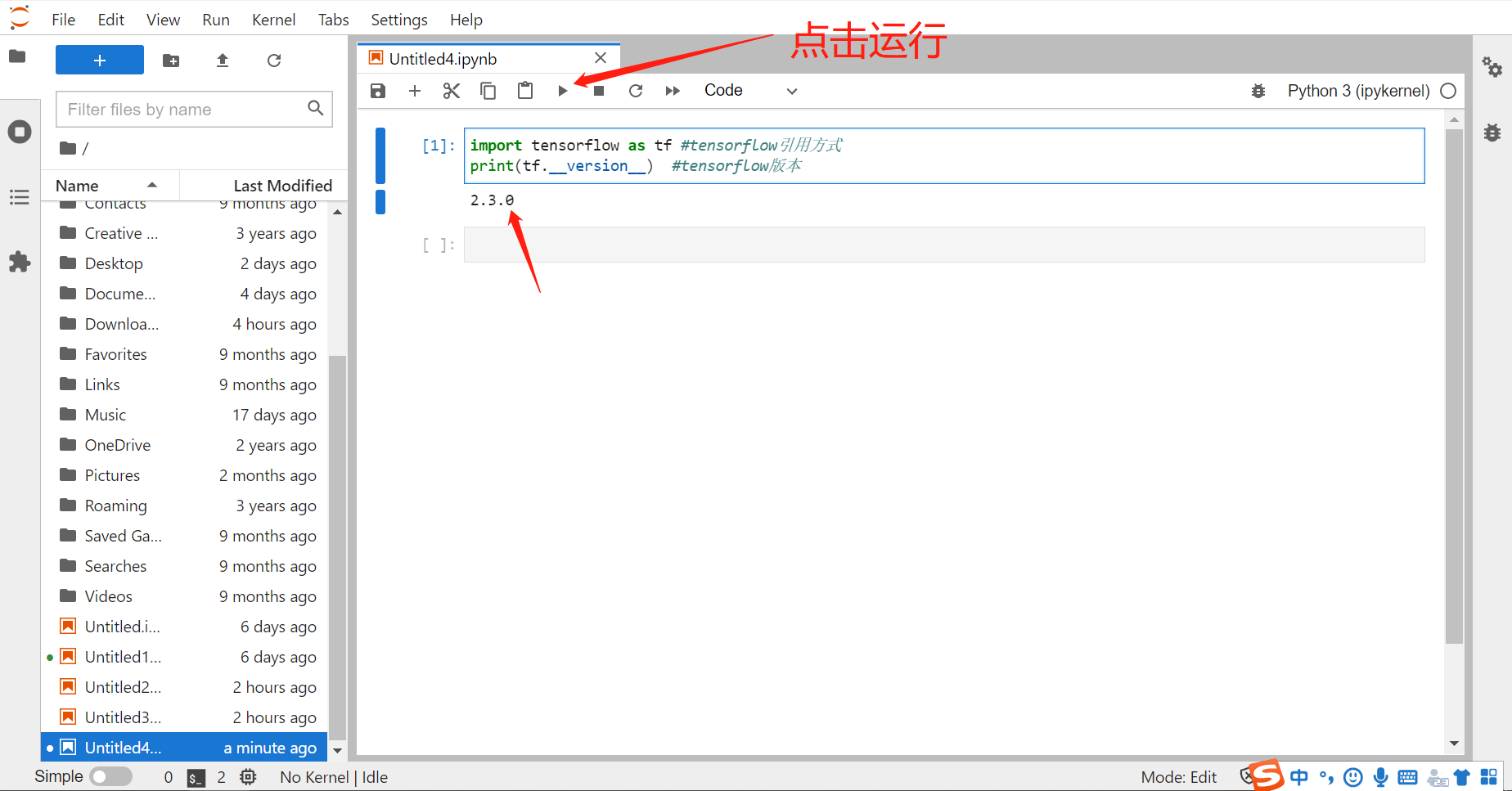Original: https://blog.csdn.net/thy0000/article/details/122783136
Author: 萝北村的枫子
Title: tensorflow安装步骤（CPU版本，Anaconda环境下，Windows10）

## 论文阅读 （三）BERT学习

【来自自评论区】 1.摘要（Abstract）：与别的文章的区别是什么？效果有多好？主要与ELMO和GPT对比 2.引言（Introduction）：语言模型的简单介绍；摘要第一段的扩充；主要想法；如...

## OpenCV-Python实战（番外篇）——利用 KNN 算法识别手写数字

OpenCV-Python实战（番外篇）——利用 KNN 算法识别手写数字 * - 前言 - 手写数字数据集 MNIST 介绍 - 基准模型——利用 KNN 算法识别手写数字 - 改进模型1——参数 ...

## slam原理介绍和经典算法

1.传统slam局限性 slam算法假设的环境中的物体都是处于静态或者低运动状态的，然而，现实世界是复杂多变的，因此这种假设对于应用 环境有着严格的限制，同时影响视觉slam系统在实际场景中的应用性。...